# Class 10 Mathematics: CBSE Sample Question Paper (2020-21) (Basic) - 1 Notes | Study CBSE Sample Papers For Class 10 - Class 10

## Class 10: Class 10 Mathematics: CBSE Sample Question Paper (2020-21) (Basic) - 1 Notes | Study CBSE Sample Papers For Class 10 - Class 10

The document Class 10 Mathematics: CBSE Sample Question Paper (2020-21) (Basic) - 1 Notes | Study CBSE Sample Papers For Class 10 - Class 10 is a part of the Class 10 Course CBSE Sample Papers For Class 10.
All you need of Class 10 at this link: Class 10

Class X
Mathematics – Basic
Sample Question Paper 2020-21
Max. Marks: 80
Duration: 3 hrs.

General Instructions

1. This question paper contains two parts A and B.
2. Both Part A and Part B have internal choices.
Part – A
1. It consists of two sections - I and II.
2. Section I has 16 questions. Internal choice is provided in 5 questions.
3. Section II has four case study-based questions. Each case study has 5 case-based sub-parts. An examinee is to attempt any 4 out of 5 sub-parts.
Part – B
1. Question No 21 to 26 are Very short answer type questions of 2 marks each.
2. Question No 27 to 33 are Short Answer Type questions of 3 marks each.
3. Question No 34 to 36 are Long Answer Type questions of 5 marks each.
4. Internal choice is provided in 2 questions of 2 marks, 2 questions of 3 marks and 1 question of 5 marks.

Part - A

Section - I

Q.1. Express 156 as the product of primes. (1 Mark)
Ans. 156 = 22 × 3 × 13

Q.2. Write a quadratic polynomial, sum of whose zeroes is 2 and product is – 8.  (1 Mark)
Ans.
Quadratic polynomial is given by: x2 – (a +b) x + ab
⇒ x2 – 2x – 8

Q.3. Given that HCF (96, 404) is 4, find the LCM (96, 404).  (1 Mark)
Ans.
HCF × LCM = product of two numbers, HCF = 4OR

State the Fundamental Theorem of Arithmetic.  (1 Mark)
Ans. Every composite number can be expressed (factorized) as a product of primes, and this factorization is unique, apart from the order in which the factors occur.

Q.4. On comparing the ratios of the coefficients, find out whether the pair of equations x – 2y = 0 and 3x + 4y – 20 = 0 is consistent or inconsistent.  (1 Mark)
Ans.
x – 2y = 0 and 3x + 4y – 20 = 0
∵ (1/3) ≠ (-2/4)
As, a1 / a2 ≠ b1 / bis one condition for consistency.
Therefore, the pair of equations is consistent.

Q.5. If a and b are co-prime numbers, then find the HCF (a, b).  (1 Mark)
Ans.
Since, 1 is the only common factor of co-prime, numbers.
Thus, HCF (a, b) = 1.

Q.6. Find the area of a sector of a circle with radius 6 cm if angle of the sector is 60°. (Take π = 22 / 7)   (1 Mark)
Ans.
θ = 60°
Area of sector =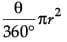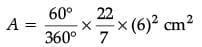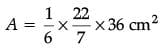= 18.86 cm2

OR

A horse tied to a pole with a 28 m long rope. Find the perimeter of the field where the horse can graze. (Take π = 22 / 7)  (1 Mark)
Ans. The horse can graze in the field which is a circle of a radius of 28 cm.
So, required perimeter = 2πr = 2.π (28) cm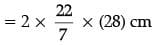= 176 cm.

Q.7. In the given fig. DE || BC, ∠ADE = 70° and ∠BAC = 50°, then angle ∠BCA = ______.   (1 Mark)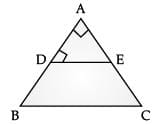Ans.
By converse of Thale’s theorem DE || BC
Given ∠BAC = 50°,
∠ABC + ∠BAC +∠BCA =180° (Angle sum prop. of triangles)
⇒ 70° + 50° + ∠BCA = 180°
⇒ ∠BCA = 180° – 120° = 60°

OR

In the given figure, AD = 2 cm, BD = 3 cm, AE = 3.5 cm and AC = 7 cm. Is DE parallel to BC?  (1 Mark)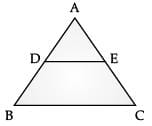Ans. EC = AC – AE = (7 – 3.5) cm = 3.5 cm
AD / BD =  2 / 3 and AE / EC = 3.5 / 3.5 = 1 / 1
So, AD / BD ≠ AE / EC
Hence, By converse of Thale’s Theorem, DE is not Parallel to BC.

Q.8. The cost of fencing a circular field at the rate of ₹ 24 per metre is ₹ 5280. Find the radius of the field.  (1 Mark)
Ans.
Length of the fence = Total cost / Rate = 5280/24 = 220 m
So, length of fence = Circumference of the field
∴ 220 m = 2πr = 2 × 22/7 x r
So,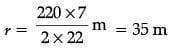Q.9. A tree breaks due to a storm and the broken part bends so that the top of the tree touches the ground where it makes an angle of 30°. The distance between the foot of the tree to the point where the top touches the ground is 8 m. Find the height of the tree from where it is broken.  (1 Mark)
Ans.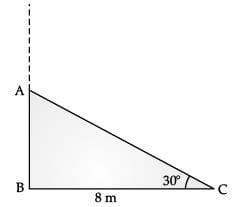tan 30 ° = AB / BC
i.e. 1/√3 = AB / 8
⇒ AB = 8 / √3
The height from where it is broken is 8/√3 metres.

Q.10. If the perimeter and the area of a circle are numerically equal, then find the radius of the circle.  (1 Mark)
Ans.
Perimeter = Area
i.e. 2πr = πr2
⇒ r = 2 units

Q.11. Write the empirical relationship among mean, median and mode.  (1 Mark)
Ans.
3 median = mode + 2 mean

Q.12. To divide a line segment BC internally in the ratio 3 : 5, we draw a ray BX such that ∠CBX is an acute angle. What will be the minimum number of points to be located at equal distances, on ray BX?  (1 Mark)
Ans.
8

Q.13. For what values of p does the pair of equations 4x + py + 8 = 0 and 2x + 2y + 2 = 0 has unique solution?  (1 Mark)
Ans.
a1 / a2 ≠ b1 / b2 is the condition for the given pair of equations to have unique solution.
4 / 2 ≠ p / 2
⇒ p ≠ 4
Therefore, for all real values of p except 4, the given pair of equations will have a unique solution.

OR

What type of straight lines will be represented by the system of equations 2x + 3y = 5 and 4x + 6y = 7?  (1 Mark)
Ans. Here, a1 / a2 = 2 / 4 = 1 / 2
b1 / b2 = 3 / 6 = 1 / 2 and c1 / c2 = 5 / 7
1 / 2 = 1 / 2 ≠ 5 / 7
a1 / a2 = b1 / b2 ≠ c1 / c2 is the condition for which the given system of equations will represent parallel lines.
So, the given system of linear equations will represent a pair of parallel lines.

Q.14. A bag contains 3 red balls and 5 black balls. A ball is drawn at random from the bag. What is the probability that the ball drawn is red?  (1 Mark)
Ans.
No. of red balls = 3, No. of black balls = 5
Total number of balls = 5 + 3 = 8
Probability of red balls = 3 / 8

OR

A die is thrown once. What is the probability of getting a prime number?  (1 Mark)
Ans. Total no of possible outcomes = 6
There are 3 Prime numbers, 2, 3, 5.
So, the probability of getting a prime number is 3 / 6.

Q.15. A tower stands vertically on the ground. From a point on the ground, which is 15 m away from the foot of the tower, the angle of elevation of the top of the tower is found to be 60°. Find the height of the tower.  (1 Mark)
Ans.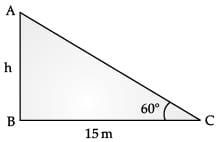tan 60° = h / 15
i.e. √3 = h / 15
⇒ h = 15√3

Q.16. Probability of an event E + Probability of the event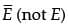is, _______.
Ans.
1

Section - II

Case study-based questions are compulsory. Attempt any four subparts of each question. Each subpart carries 1 mark.

Q.17.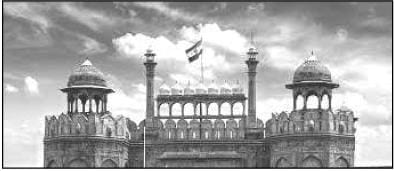Mathematics teacher of a school took her 10th standard students to show Red fort. It was a part of their Educational trip. The teacher had interest in history as well. She narrated the facts of Red fort to students. Then the teacher said in this monument one can find combination of solid figures. There are 2 pillars which are cylindrical in shape. Also 2 domes at the corners which are hemispherical. 7 smaller domes at the centre. Flag hoisting ceremony on Independence Day takes place near these domes.
(i) How much cloth material will be required to cover 2 big domes each of radius 2.5 metres? (Take π = 22/7)  (1 Mark)
(a) 75 m2

(b) 78.57 m2
(c) 87.47 m2
(d) 25.8 m2
Ans. (b)
Solution: Cloth material required = 2 × S.A. of hemispherical dome
Cloth material required = 2 × 2πr= 2 × 2 × 22 / 7 × (2.5)2 m2
Cloth material required = 78.57 m2

(ii) Write the formula to find the volume of a cylindrical pillar.    (1 Mark)
(a) πr2h
(b) πrl
(c) πr(l + r)
(d) 2πr
Ans.
(a)
Solution: Volume of a cylindrical pillar = πr2h

(iii) Find the lateral surface area of two pillars if height of the pillar is 7 m and radius of the base is 1.4 m.    (1 Mark)
(a) 112.3 cm2
(b) 123.2 m2
(c) 90 m2
(d) 345.2 cm2

Ans. (b)
Solution: Lateral surface area = 2 × 2πrh
Lateral surface area = 4 × 22/7 × 1.4 × 7 m= 123.2 m2

(iv) How much is the volume of a hemisphere if the radius of the base is 3.5 m?  (1 Mark)
(a) 85.9 m3
(b) 80 m2
(c) 98 m3
(d) 89.83 m3
Ans. (d)
Solution: Volume of hemisphere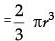Volume of hemisphere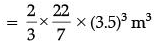= 89.83 m3

(v) What is the ratio of sum of volumes of two hemispheres of radius 1 cm each to the volume of a sphere of radius 2 cm ?  (1 Mark)
(a) 1:1
(b) 1:8
(c) 8:1
(d) 1:16
Ans. (b)
Solution:
Sum of the volumes of two hemispheres of radius 1 cm each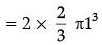Volume of sphere of radius 2 cm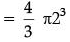So, required ratio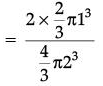= 1:8

Q.18. Class X students of a secondary school in Krishnagar have been allotted a rectangular plot of land for gardening activity. Saplings of Gulmohar are planted on the boundary at a distance of 1 m from each other. There is a triangular grassy lawn in the plot as shown in fig. The students are to sow seeds of flowering plants on the remaining area of the plot.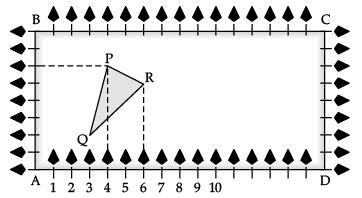Considering A as origin, answer question (i) to (v):
(i) What are the coordinates of A?  (1 Mark)
(a) (0, 1)
(b) (1, 0)
(c) (0, 0)
(d) (–1, –1)
Ans. (c)

(ii) What are the coordinates of P?  (1 Mark)
(a) (4, 6)
(b) (6, 4)
(c) (4, 5)
(d) (5, 4)
Ans. (a)

(iii) What are the coordinates of R?  (1 Mark)
(a) (6, 5)
(b) (5, 6)
(c) (6, 0)
(d) (7, 4)
Ans. (a)

(iv) What are the coordinates of D?  (1 Mark)
(a) (16, 0)
(b) (0, 0)
(c) (0, 16)
(d) (16, 1)
Ans. (a)

(v) What are the coordinate of P if D is taken as the origin?  (1 Mark)
(a) (12, 2)
(b) (– 12, 6)
(c) (12, 3)
(d) (6, 10)
Ans. (b)

Q.19. Rahul is studying at X Standard. He is making a kite to fly it on a Sunday. Few questions came to his mind while making the kite. Give answers to his questions by looking at the figure: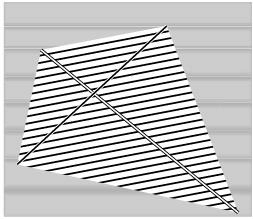(i) Rahul tied the sticks at what angles to each other?  (1 Mark)
(a) 30°
(b) 60°
(c) 90°
(d) 120°
Ans. (c)

(ii) Which is the correct similarity criteria applicable for smaller triangles at the upper part of this kite?  (1 Mark)
(a)
RHS
(b) SAS
(c) SSA
(d) AAS
Ans. (b)

(iii) Sides of two similar triangles are in the ratio 4:9. Corresponding medians of these triangles are in the ratio,  (1 Mark)
(a)
2:3
(b) 4:9
(c) 81:16
(d) 16:81
Ans. (b)

(iv) In a triangle, if the square of one side is equal to the sum of the squares of the other two sides, then the angle opposite the first side is a right angle. This theorem is called as,  (1 Mark)
(a)
Pythagoras theorem
(b) Thales theorem
(c) Converse of Thales theorem
(d) Converse of Pythagoras theorem
Ans. (d)

(v)  What is the area of the kite, formed by two perpendicular sticks of length 6 cm and 8 cm?  (1 Mark)
(a) 48 cm2
(b) 14 cm2
(c) 24 cm2
(d) 96 cm2
Ans. (c)

Q.20. Due to a heavy storm, an electric wire got bent as shown in the figure. It followed a mathematical shape. Answer the following questions below: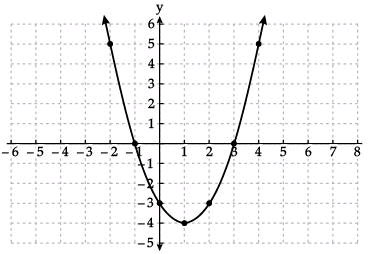(i) Name the shape in which the wire is bent  (1 Mark)
(a) Spiral
(b) ellipse
(c) linear
(d) Parabola
Ans. (d)

(ii) How many zeroes are there for the polynomial (shape of the wire)  (1 Mark)
(a)
2
(b) 3
(c) 1
(d) 0
Ans. (a)

(iii) The zeroes of the polynomial are :  (1 Mark)
(a)
–1, 5
(b) –1, 3
(c) 3, 5
(d) –4, 2
Ans. (a)

(iv) What will be the expression of the polynomial?  (1 Mark)
(a)
x2 + 2x – 3
(b) x2 – 2x + 3
(c) x2 – 2x – 3
(d) x2 + 2x + 3
Ans. (c)

(v) What is the value of the polynomial if x = – 1?  (1 Mark)
(a)
6
(b) –18
(c) 18
(d) 0
Ans. (d)

Part - B

All questions are compulsory. In case of internal choices, attempt anyone.

Q.21. Find the coordinates of the point which divides the line segment joining the points (4, – 3) and (8, 5) in the ratio 3:1 internally.  (2 Mark)
Ans. Let P (x, y) be the required point. Using section formula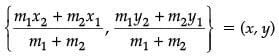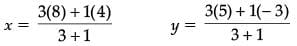x = 7, y = 3
(7, 3) is the required point

OR

Find a relation between x and y such that the point (x, y) is equidistant from the points (7, 1) and (3, 5).  (2 Mark)
Ans. Let P(x, y) be equidistant from the points A(7, 1) and B(3, 5)
Given AP = BP.  So, AP2 = BP2
(x – 7)2 + (y – 1)2 = (x – 3)2 + (y – 5)2
x2 – 14x + 49 + y2 – 2y +1 = x2 – 6x + 9 + y2 – 10y + 25
x – y = 2

Q.22. In the fig. if LM || CB and LN || CD, prove that AM / MB = AN / ND  (2 Mark)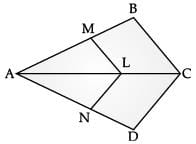Ans. By BPT, In ΔABC
AM / MB = AL / LC ....(1)
AN / ND = AL / LC ....(2)
By Equating (1) and (2), AM/MB = AN/ND

Q.23. A quadrilateral ABCD is drawn to circumscribe a circle. Prove that AB + CD = AD + BC.  (2 Mark)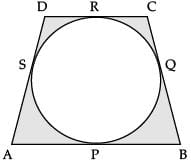Ans. To prove: AB + CD = AD + BC.

Proof: AS = AP (i) (Length of tangents from an external point to a circle are equal)
BQ = BP ...(ii)
CQ = CR ...(iii)
DS = DR ...(iv)
Adding e.q., (i), (ii), (iii), and (iv)
AS + BQ + CQ + DS = AP + BP + CR + DR
(AS + DS) + (BQ + CQ) = (AP + BP) + (CR + DR)
AD + BC = AB + CD

Q.24. Draw a line segment of length 7.8 cm and divide it in the ratio 5:8. Measure the two parts.  (2 Mark)
Ans. Steps of constructions:
(i) Draw line segment AB of length 7.8 cm.
(ii) Draw any ray AX, making an acute angle with AB.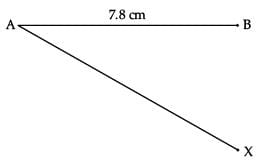(iii) Mark 13 (= 5 + 8) points A1, A2, A3, A4, ......... A13, on AX such that AA1 = A1A2 = A2A3 ........... by drawing equal arcs.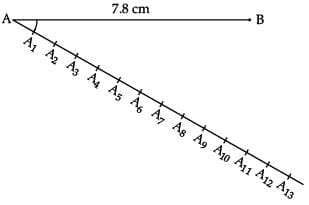(iv) Join BA13.
(v) Since we want the ratio 5 : 8, Through point A5 (m = 5), we draw a line parallel to A13B by making ∠AA5C = ∠AA13B So, we copy ∠AA13B from point A5.
Thus, AC : CB = 5 : 8.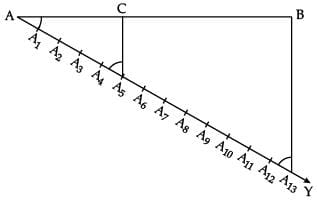On measuring AC and BC by scale, AC = 3.0 cm & BC = 4.8 cm.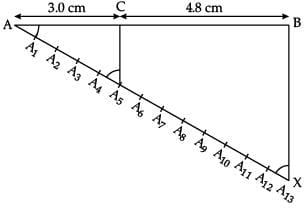Q.25. Given 15 cot A = 8, find sin A and sec A.  (2 Mark)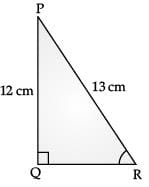Ans. 15 cot A = 8 ⇒ cot A = 8/15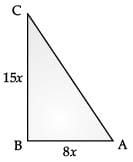Adj. / Oppo. = 8 / 15
By Pythagoras Theorem, AC2 = AB2 + BC2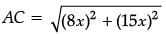= 17x
sin A = 15 / 17
sec A = 17 / 8

OR
Find tan P – cot R  (2 Mark)Ans. By Pythagoras Theorem,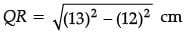= 5 cm
tan P = 5/12
cot R = 5/12
tan P – cot R = 5 / 12 - 5 / 12 = 0

Q.26. How many terms of the A. P : 9, 17, 25, ....... must be taken to give a sum 636? (2 Mark)
Ans. 9, 17, 25, .......
Sn = 636, a = 9
d = a2 – a1 = 17 – 9 = 8
Sn = (n/2) [2a +(n − 1)d]
636 = (n/2) [2 ×9+(n − 1)8]
1272 = n [18 + 8n – 8]
1272 = n [10 + 8n]
8n2 +10n – 1272 =0
4n2 + 5n – 636 = 0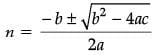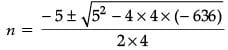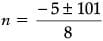n = 96 / 8, n = -106 / 8
n = 12, n = -53 / 4
n =12 (since n cannot be negative)

All questions are compulsory. In case of internal choices, attempt anyone.
Q.27. Prove that √3 is an irrational number.  (3 Mark)
Ans. Let √3 be a rational number.
Then √3 = p/q, HCF (p, q) =1
Squaring both sides
(√3)2 = (p/q)2
3 = p2/q2
3q2 = p2
3 divides p2 » 3 divides p
3 is a factor of p
Take p = 3C
3q2 = (3C)2
3q2 = 9C2
3 divides q2 » 3 divides q
3 is a factor of q
Therefore 3 is a common factor of p and q
It is a contradiction to our assumption that p / q is rational.
Hence 3 is an irrational number.

Q.28. Two tangents TP and TQ are drawn to a circle with centre O from an external point T. Prove that ∠PTQ = 2∠OPQ.  (3 Mark)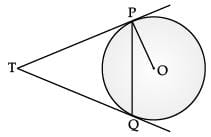Ans. Required to prove: ∠PTQ = 2∠OPQ
Proof: Let ∠PTQ = θ
Now by the theorem TP = TQ. So, TPQ is an isosceles triangle
∠TPQ = ∠TQP = ½ (180° – θ) = 90° – ½ θ
∠OPT = 90°
∠OPQ =∠OPT – ∠TPQ = 90° – (90° – ½ θ) = ½ θ = ½ ∠PTQ
∠PTQ = 2∠OPQ

Q.29. Meena went to a bank to withdraw ₹2,000. She asked the cashier to give her ₹50 and ₹100 notes only. Meena got 25 notes in all. Find how many notes of ₹50 and ₹100 she received.  (3 Mark)
Ans.
Let Meena has received x no. of ₹50 notes and y no. of ₹100 notes. So,
50x + 100y = 2000 ...(i)
x + y = 25 ...(ii)
multiply by 50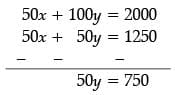y = 15
Putting value of y = 15 in equation (ii)
x + 15 = 25
x = 10
Meena has received 10 pieces ₹50 notes and 15 pieces of ₹100 notes.

Q.30. A box contains 90 discs which are numbered from 1 to 90. If one disc is drawn at random from the box, (3 Mark)
Find the probability that it bears:
(i) a two-digit number.
(ii) a perfect square number.
(iii) a number divisible by 5.

Ans. (i) 10, 11, 12… 90 are two-digit numbers. There are 81 numbers.
So, Probability of getting a two-digit number = 81/90 = 9/10
(ii) 1, 4, 9, 16, 25, 36, 49, 64, 81 are perfect squares.
So, the probability of getting a perfect square number = 9/90 = 1/10
(iii) 5, 10, 15…. 90 are divisible by 5. There are 18 outcomes.
So, the Probability of getting a number divisible by 5 = 18 / 90 = 1 / 5

OR

One card is drawn from a well-shuffled deck of 52 cards. Find the probability of getting  (3 Mark)
(i) A king of red colour.
(iii) The queen of diamonds.

Ans. (i) P (King of red colour) = 2 / 52 = 1 / 26
(ii) P (a spade) = 13 / 52 = 1 / 4
(iii) P (a queen of diamonds) = 1 / 52

Q.31. Metallic spheres of radii 6 cm, 8 cm and 10 cm respectively are melted to form a solid sphere. Find the radius of the resulting sphere.  (3 Mark)
Ans. r1 = 6 cm, r2 = 8 cm, r3 = 10 cm
Volume of sphere =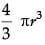Volume of the resulting sphere = Sum of the volumes of the smaller spheres.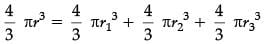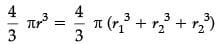⇒ r3 = 63 + 83 + 10= 1728
⇒ r = ∛1728 = 12 cm
Therefore, the radius of the resulting sphere is 12 cm.

Q.32. Prove that  (3 Mark)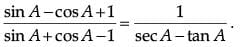Ans.
L.H.S. divide numerator and denominator by cos A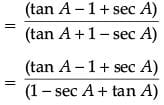We know that: 1 + tan2A = sec2A Or 1 = sec2A – tan2A
= (sec A + tan A)(sec A – tan A)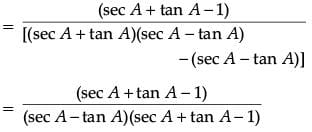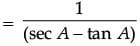Hence proved.

Q.33. A motorboat whose speed in still water is 18 km/h, takes 1 hour more to go 24 km upstream than to return downstream to the same spot. Find the speed of the stream.
Ans. Given: Speed of boat = 18 km/hr, Distance = 24 km
Let x be the speed of stream.
Let t1 and t2 be the time for upstream and downstream.
As we know that: speed = distance / time
⇒ time = distance / speed
For upstream:
Speed = (18  x) km/hr, Distance = 24 km
Time = t1
Therefore, t1 = 24 / 18 − x
For downstream:
Speed = (18 + x) km/hr, Distance = 24 km
Time = t2
Therefore, t2 = 24 / 18 − x
According to the question:
t1 = t2 + 1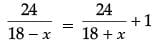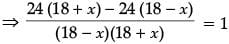⇒ 48x = (18 − x)(18 + x)
48x = 324 + 18x − 18x − x2
⇒ x2 + 48x − 324 = 0
⇒ x2 + 54x − 6x − 324 = 0
x(x + 54)  6(x + 54) = 0
(x + 54)(x − 6) = 0
x = −54 or x = 6
Since speed cannot be negative.
⇒ x = − 54 will be rejected
x = 6
Thus, the speed of stream is 6 km/hr.
OR
Find two consecutive odd positive integers, sum of whose squares is 290.

Ans. Let one of the odd positive integer be x then the other odd positive integer is x + 2 their sum of squares = x2 +(x + 2)2 = x+ x+ 4x + 4
⇒ 2x2 + 4x + 4
Given that their sum of squares = 290
⇒ 2x2 + 4x + 4 = 290 1
⇒ 2x2 + 4x = 290 – 4 = 286
⇒ 2x2 + 4x – 286 = 0
⇒ 2(x2 + 2x – 143) = 0
⇒ x2 + 2x – 143 = 0
⇒ x2 + 13x – 11x – 143 = 0 1
⇒ x(x + 13) – 11(x + 13) = 0
⇒ (x – 11)(x + 13) = 0
⇒ (x – 11) = 0, (x + 13) = 0
Therefore, x = 11 or – 13
According to question, x is a positive odd integer. Hence, We take positive value of x
So, x = 11 and (x + 2) = 11 + 2 = 13
Therefore, the odd positive integers are 11 and 13.

All questions are compulsory. In case of internal choices, attempt any one.
Q.34. The angles of depression of the top and bottom of the 8 m tall building from the top of a multi-storied building are 30° and 45°, respectively. Find the height of the multi-storied building and the distance between the two buildings.
Ans.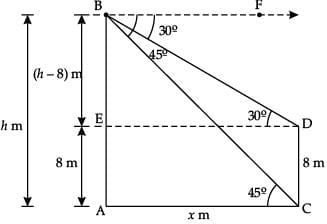Let AB and CD be the multi-storied building and the building respectively.
Let the height of the multi-storied building = h m and the distance between the two buildings = x m.
AE = CD = 8 m [Given]
BE = AB – AE = (h – 8) m
and AC = DE = x m [Given]
Also, ∠FBD = ∠BDE = 30° ( Alternate angles)
∠FBC = ∠BCA = 45° (Alternate angles)
Now, In Δ ACB,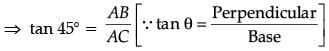⇒ 1 = h / x
⇒ x = h ...(i)
In Δ BDE,
⇒ tan 30° = BE / ED
⇒ 1 / √3 = h - 8 / x
⇒ x = √3 ( h − 8) ...(ii)
F (i) and (ii), we get,
h = √3h − 8√3
√3h −h = 8√3
h (√3 −1) = 8√3
h = 8√3 / √3 - 1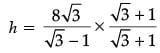h = 4√3 (√3 +1)
h = 12 + 4√3 m
Distance between the two building
x = (12 + 4√3) m [From (i)]

OR

A 1.2 m tall girl spots a balloon moving with the wind in a horizontal line at a height of 88.2 m from the ground. The angle of elevation of the balloon from the eyes of the girl at any instant is 60°. After some time, the angle of elevation reduces 30°. Find the distance travelled by the balloon during the interval.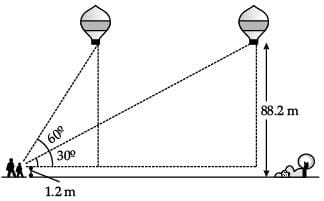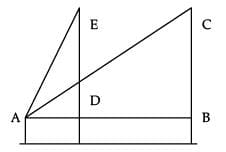Ans. From the figure, the angle of elevation for the first position of the balloon ∠EAD = 60° and for the second position ∠BAC = 30°.
The vertical distance:
ED = CB = 88.2 – 1.2 = 87 m.
Let, AD = x m and AB = y m.
tan 60° = DE / AD i.e. √3 = (87/x)
⇒ x = 87 / √3
In right ΔABC:
tan 30° = BC / AB i.e. (1/√3) = (87 / y)
⇒ y = 87√3 ...(ii)
Subtracting (i) and (ii)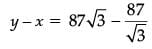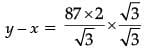Hence, the distance travelled by the balloon is equal to BD
y – x = 58√3m.

Q.35. The pth, qth and rth terms of an A.P. are a, b and c respectively.
Show that a(q – r) + b(r – p) + c(p – q) = 0  (5 Mark)

Ans. Let A be the first term and D be the common difference of A.P.
Tp = a = A + (p − 1) D = (A  D) + pD ...(1)
Tq = b = A + (q  1) D = (A  D) + qD ...(2)
Tr = c = A + (r  1) D = (A  D) + rD ...(3)
Here we have got two unknowns A and D which are to be eliminated.
On multiply (1), (2) and (3) by q − r, r − p and p − q respectively
a (q – r) = (A – D) (q – r) + Dp (q – r)
b (r – p) = (A – D) (r – p) + Dq (r – p)
c (p – q) = (A – D) (p – q) + Dr (p – q)
a (q  r) + b (r  p) + c (p  q)
= (A  D) [q  r + r  p + p  q] + D [p (q  r) + q (r  p) + r (p − q)]
= (A – D ) (0) + D [pq – pr + qr – pq + rp – rq)
= 0.

Q.36. A survey regarding the heights in (cm) of 51 girls of class X of a school was conducted and the following data was obtained. Find the median height and the mean using the formulae.  (5 Mark)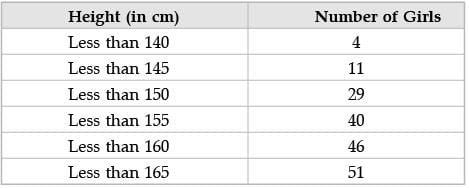Ans.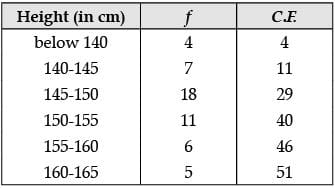N = 51 ⇒ N / 2 = 51 / 2 = 25.5
As 29 is just greater than 25.5, therefore median class is 145 – 150.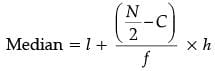Here, l = lower limit of median class =145 C = C.F. of the class preceding the median class = 11
h = higher limit - lower limit = 150 - 145 = 5
f = frequency of median class =18
∴ Median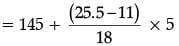=149.03
Mean by direct method,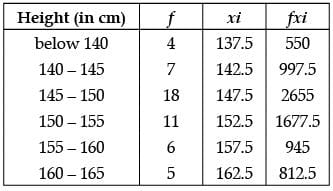Mean = fx / N = 7637.5/51 = 149.75.

The document Class 10 Mathematics: CBSE Sample Question Paper (2020-21) (Basic) - 1 Notes | Study CBSE Sample Papers For Class 10 - Class 10 is a part of the Class 10 Course CBSE Sample Papers For Class 10.
All you need of Class 10 at this link: Class 10Use Code STAYHOME200 and get INR 200 additional OFF

## CBSE Sample Papers For Class 10

174 docs|7 tests

Track your progress, build streaks, highlight & save important lessons and more!

,

,

,

,

,

,

,

,

,

,

,

,

,

,

,

,

,

,

,

,

,

;